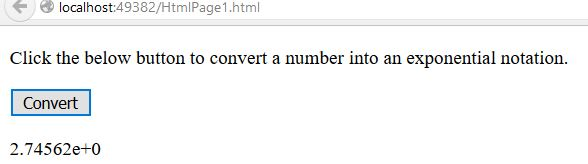# Exponential format in JavaScript

#### Exponential

Exponential is an mathematical function and refers to the number of times a number is multiplied by itself. For example 2 to the power of 4, mathematically that can be written as 24. That means 2 is multiplies by itself 4 times.

24 = 2 X 2 X 2 X 2 =16.

the exponential is in the form of

f(b) = ab

NOTE: The value of e=10, where e+0 is nothing but the 10 power 0, the value of e+0 is 1.

In JavaScript, by using `toExponential()` method we can convert a number into an exponential notation.

### Converting number 2.74562 to exponential notaion.

```<p>Click the below button to convert a number into an exponential notation.</p>
<input type="button" value="Convert" onclick="Function()" />
<p id="myId"></p>

<script>
function Function() {
var A = 2.74562;
var R = A.toExponential();
document.getElementById("myId").innerHTML = R;
}
</script>```

In the above code snippet we have given `Id` as "`myId`"to the second `<p>` element in the HTML code. There is a Function() in the`<script>`block which is connected to the Onclick of the HTML button. We need to convert a number into an exponential(scientific) notation, for that we are using `toExponential()` method. Onclick of the button "Convert" fires the Function() in the `<script>` block at the same time `toExponential()` method convert a number into an exponential notation and gives the output.

OUTPUT### Converting only 2 (After decimal point) digits into exponential in JavaScript

```<p>Click the below button to convert 2 numbers into an exponential notation.</p>
<input type="button" value="Convert" onclick="Function()" />
<p id="myId"></p>

<script>
function Function() {
var A = 2.74562;
var R = A.toExponential(2);
document.getElementById("myId").innerHTML = R;
}
</script>
```

Converting only 2 digit decimal number to exponential notation

We need convert the decimal number to two digit exponential decimal number, for that we are using `toExponential(2) `method. Onclick of the button "Convert" in the HTML code fires the Function() in the `<script>` block at the same time `toExponential()` method convert the deimal into exponential form and return the output.

OUTPUT### Converting 2.74562 to approximate nearer single digit exponential value

```<p>Click the below button to convert decimal into approximate exponential notation.</p>
<input type="button" value="Convert" onclick="Function()" />
<p id="myId"></p>

<script>
function Function() {
var A = 2.74562;
var R = A.toExponential(0);
document.getElementById("myId").innerHTML = R;
}
</script>
```

Converting to approximate exponential value

We need to convert the decimal value to approximate nearer single digit exponential form number, for that we are using `toExponential(0)`. Onclick of the button gives the approximate exponential form single digit number.

OUTPUTViews: 5803 | Post Order: 88